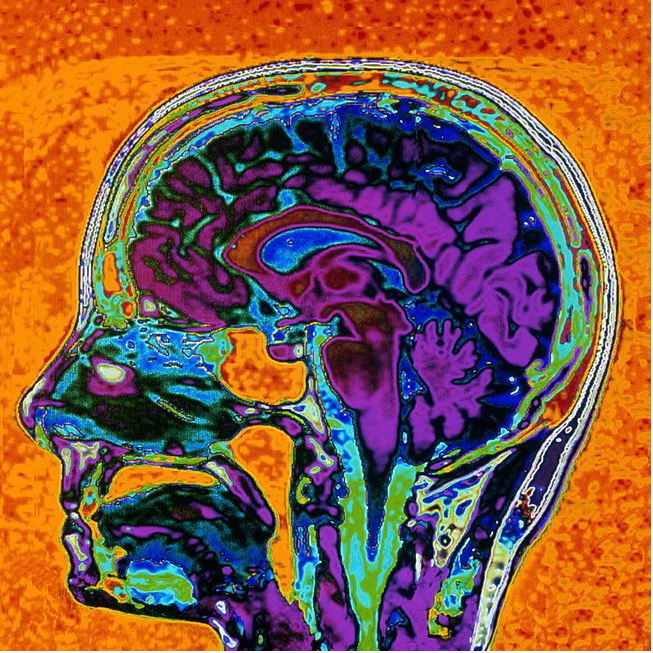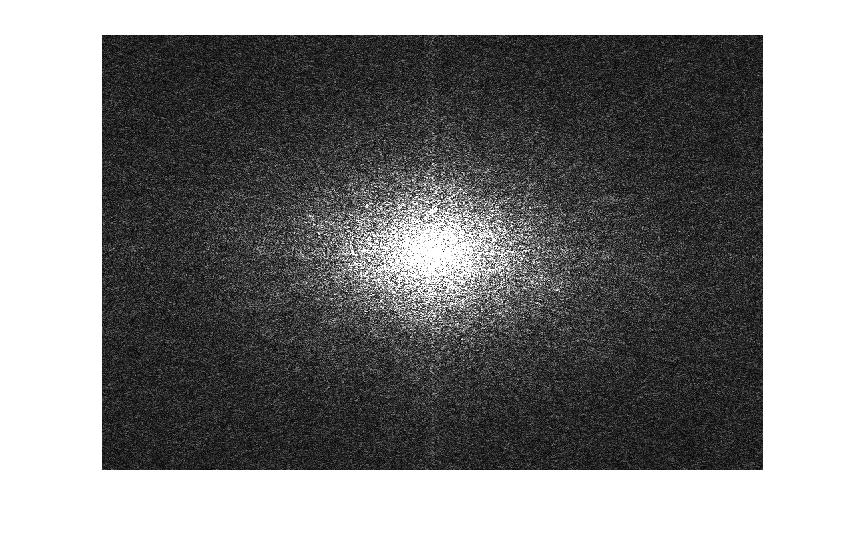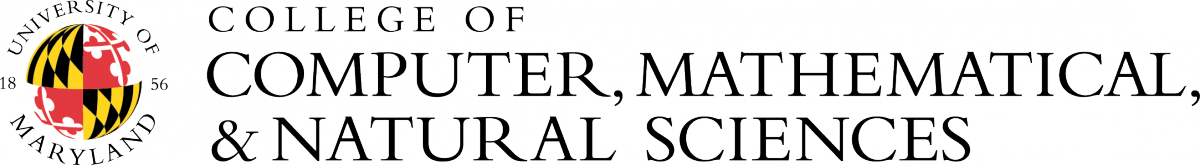Home> Research Activities> DFT> Introduction

Introduction

Publications
Software

### Discrete Fourier Transform

The discrete Fourier transform (DFT) is a multidisciplinary formula that relates the spectrum of a function or signal described by a finite sequence of function values. Among its many applications, the DFT is used for audio and image processing, for solving partial differential equations, and for facilitating convolution and polynomial multplication.

For a function

the discrete Fourier transform $F$ of $f$ is defined by
The calculation of this sum takes $N$ computational steps, making the computation of the $N$ Fourier coefficients an order $N2$ computation, that is computationally cumbersome for large N. For example, a 720p image frame f requires on the order 239 computational steps to find all of its Fourier coefficients, F[n].

Thus, in the early days of computing, the usefulness of formula (1) was minimal. However, in 1965 James Cooley (a mathematician for IBM) and John Tukey (a mathematician at Princeton and Bell labs) wrote a paper describing what would become known as the fast Fourier transform (FFT) algorithm, that reduced the computational cost to the order of Nlog(N) steps. While it soon became well-known that the algorithm existed in different forms and applications dating back to Gauss, the Cooley-Tukey paper brought Fourier analysis to the forefront of scientific computing.

Since the Cooley-Tukey paper, there have been variations and improvements to the FFT algorithm, including the fastest Fourier transform in the west (FFTW) and the sparse Fourier transform. The publications section of this site is partitioned into a history section, as well as listing references for these variations and improvements. The software section contains links to several open source implementations of these algorithms.An image of an MRI, and a representation of the modulus of its Fourier transform.

1. [E.O. Brigham, and R.E. Morrow, The fast Fourier transform, Spectrum, IEEE 4.12 (1967), 63-70.]### Stay ConnectedNorbert Wiener Center
Department of Mathematics
University of Maryland
College Park, MD 20742
Phone: (301) 405-5158
The Norbert Wiener Center is part of the College of Computer, Mathematical, and Natural Sciences.